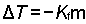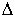Freezing Point Depression

Depression of the Freezing Point of a Solvent

We need two pieces of information to calculate the depression of the freezing point of the solvent in a solution containing a nonvolatile nonelectrolyte:

• The molal concentration, m, of the solute in the solution (Sometimes it may be necessary to calculate this concentration from other information.)
• The freezing point depression constant, Kf, for the solvent.

We use the following equation to calculate a freezing point depression. (Note: This equation assumes the freezing point depression constant has a positive value.)Freezing Point of a Solution

The freezing point of the solvent in a solution containing a nonvolatile nonelectrolyte, Tsolution, may be found from the following information:

• The freezing point of the pure solvent, Tpure solvent.
• The freezing point depression,T.Note: The freezing point of a solution will always be lower than the freezing point of the pure solvent.